# How to Get Position of First Partial Match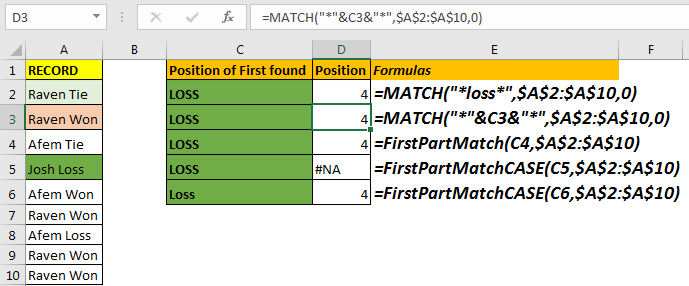In this article, we will try to get position of first partial match in a range.
As we know that MATCH function returns the index or position of first match in a range. Hence, it is obvious to use this function to get the  position of first match in a range. Since, it supports the wildcard operators, we can also use MATCH to do partial matches.

Once we get the position of first match position, we can do various things. Like retrieving that value or adjacent or non adjacent value using INDEX function,, or making a dynamic function. It depends on your need and creativity.
Generic Formula for First Found Partial MATCH
For hardcode string:

=MATCH("*str*",range,0)

For cell reference:

=MATCH("*"&cell&"*",range,0)

Str: it is the text or string that you want to partially match in range. It can be any thing, a cell or a hardcoded string.
Range: it is the range in which you will look for str.
0: It is the parameter for exact match. You can use FALSE too.

Note that we used to * (astrisks) in front and end of string str. This is the wildcard operator for matching any value having any string before and after it..

Let’s see an example.

Here I have a record of Win, Loss, and Tie. We want to get first position of Win, Loss, and Tie.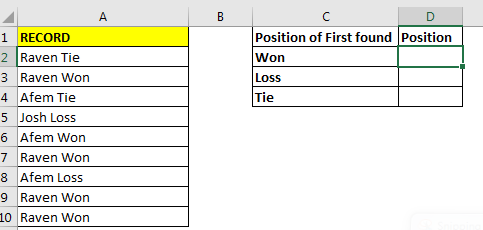So, if I hard code then formula for finding first position of partial match in each cell will be:

=MATCH("*won*",A2:A10,0)
=MATCH("*loss*",A2:A10,0)
=MATCH("*tie*",A2:A10,0)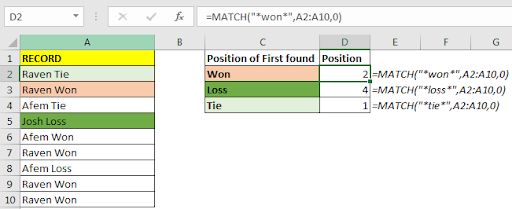=MATCH("*"&C2&"*",\$A\$2:\$A\$10,0)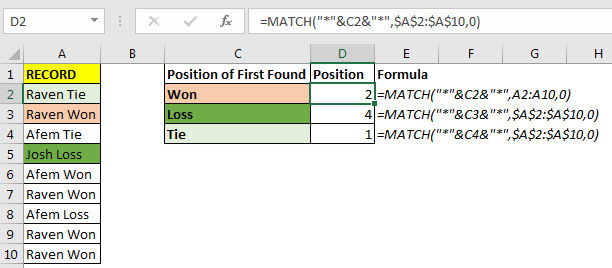How it works
It simply uses the functionality of excel to do partial matches. MATCH looks for any string that contains the provided string between * (asterisks) and returns the first found position.
Find Position of First Partial Match in Range using FirstPartMatch VBA Function
If you copy below vba code in vba module in excel, you can use this function to get first position of partial match. For above example, just write this formula:

=FirstPartMatch(C2,\$A\$2:\$A\$10)

You’ll get the position of first match. Like this.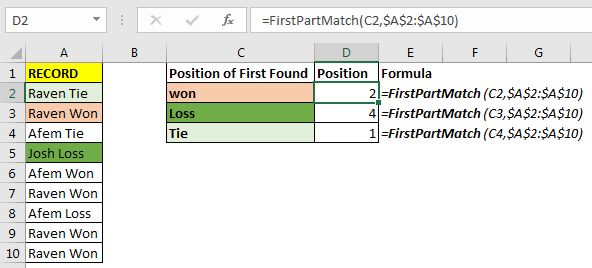How It Works

First argument is the string you want to search for partial match.
The second argument is the range in which you want to get position of your string.

=FirstPartMatch(str,range)

To use this formula for partial matching, copy below code in VBA module.

```Function FirstPartMatch(str As String, rng As Range)
Dim tmp, position As Long
position = 0
tmp = 0

For Each cll In rng
tmp = tmp + 1
If InStr(1, LCase(cll.Value2), LCase(str)) > 0 Then
position = tmp
Exit For
End If
Next cll

If position Then
FirstPartMatch = position
Else
FirstPartMatch = "#NA"
End If

End Function
```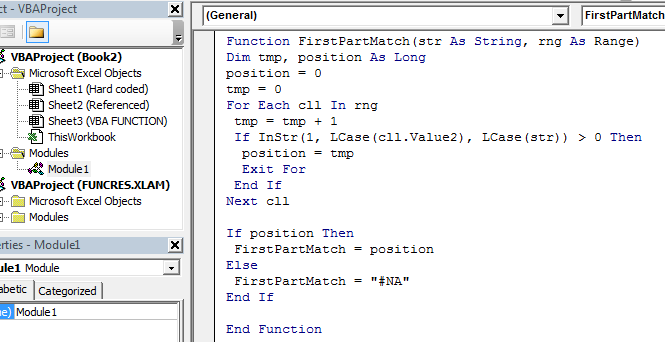Case Sensitive Partial Match for Position

Above user defined function for finding first partial match will not be case sensitive. If you want it to be case sensitive, remove Lcase function from line 7.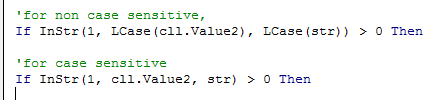Then the code for case sensitive will be:

```Function FirstPartMatchCASE(str As String, rng As Range)
Dim tmp, position As Long
position = 0
tmp = 0

For Each cll In rng
tmp = tmp + 1
If InStr(1, cll.Value2, str) > 0 Then
position = tmp
Exit For
End If
Next cll

If position Then
FirstPartMatchCASE = position
Else
FirstPartMatchCASE = "#NA"
End If

End Function

```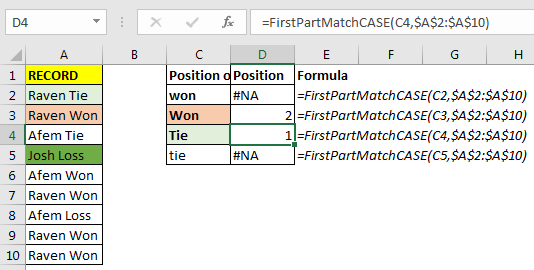You can see that “won” and “tie” in this case are not found. Since there is no lower case won or tie.

So yeah guys, these are the ways to find the position of first partial match in excel. I told you you can find frist partial match position using predefined MATCH function and using user defined match function. If you have any other ideas or question, do share with us here in the comments section below.

Related Articles:

How to Use MATCH Function in Excel

How to Use INDEX Function in Excel

Vlookup Top 5 Values with Duplicate Values Using INDEX-MATCH in Excel

Popular Articles:

The VLOOKUP Function in Excel

COUNTIF in Excel 2016

How to Use SUMIF Function in Excel

Terms and Conditions of use

The applications/code on this site are distributed as is and without warranties or liability. In no event shall the owner of the copyrights, or the authors of the applications/code be liable for any loss of profit, any problems or any damage resulting from the use or evaluation of the applications/code.